# 迹忆客专注技术分享

## Consistent Hashing算法入门及PHP代码实现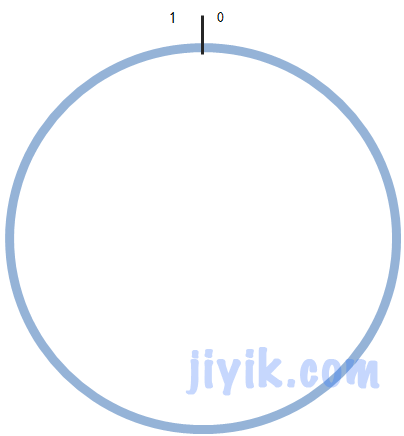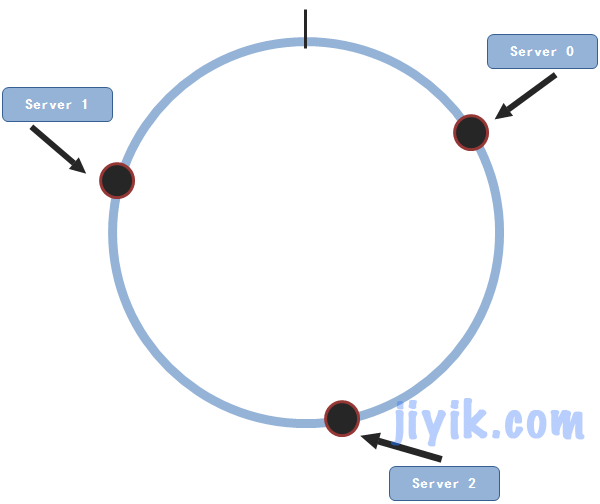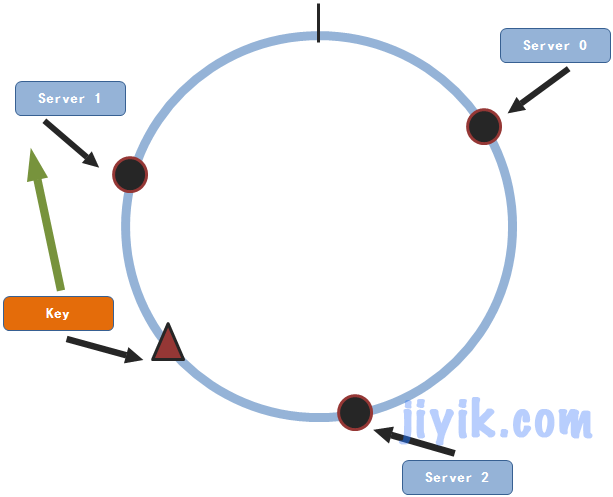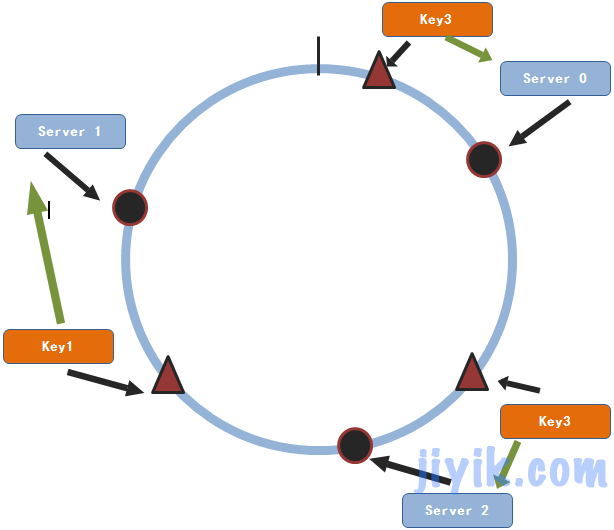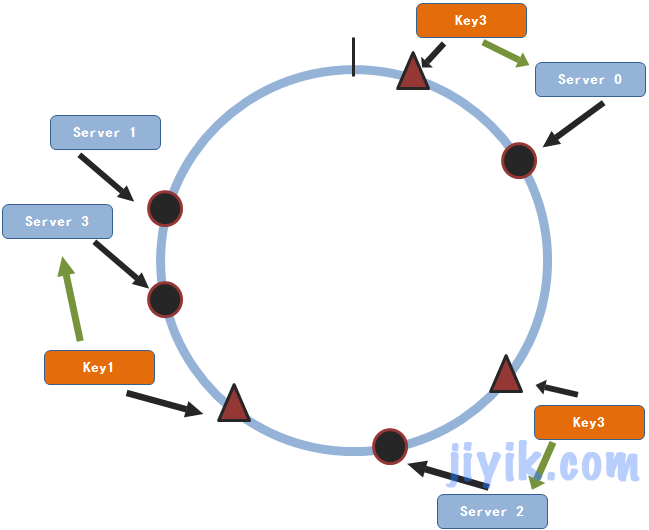``````\$nodes = array('192.168.5.201','192.168.5.102','192.168.5.111');
\$keys = array('onmpw', 'jiyi', 'onmpw_key', 'jiyi_key', 'www','www_key','key1');
\$buckets = array(); //节点的hash字典
\$maps = array(); //存储key和节点之间的映射关系
/**
* 生成节点字典 —— 使节点分布在单位区间[0,1)的圆上
*/
foreach( \$nodes as \$key) {
\$crc = crc32(\$key)/pow(2,32);            // CRC値
\$buckets[] = array('index'=>\$crc,'node'=>\$key);
}

/*
* 根据索引进行排序
*/
sort(\$buckets);
/*
* 对每个key进行hash计算，找到其在圆上的位置
* 然后在该位置开始依顺时针方向找到第一个服务节点
*/
foreach(\$keys as \$key){
\$flag = false; //表示是否有找到服务节点
\$crc = crc32(\$key)/pow(2,32);//计算key的hash值
for(\$i = 0; \$i < count(\$buckets); \$i++){

if(\$buckets[\$i]['index'] > \$crc){
/*
* 因为已经对buckets进行了排序
* 所以第一个index大于key的hash值的节点即是要找的节点
*/
\$maps[\$key] = \$buckets[\$i]['node'];
\$flag = true;
break;
}
}
if(!\$flag){
//没有找到，则使用buckets中的第一个服务节点
\$maps[\$key] = \$buckets['node'];
}
}
foreach(\$maps as \$key=>\$val){
echo \$key.'=>'.\$val,"<br />";
}``````

onmpw=>192.168.5.102
jiyi=>192.168.5.201
onmpw_key=>192.168.5.201
jiyi_key=>192.168.5.102
www=>192.168.5.201
www_key=>192.168.5.201
key1=>192.168.5.111

``\$nodes = array('192.168.5.201','192.168.5.102','192.168.5.111','192.168.5.11');``

onmpw=>192.168.5.102
jiyi=>192.168.5.201
onmpw_key=>192.168.5.11
jiyi_key=>192.168.5.102
www=>192.168.5.201
www_key=>192.168.5.201
key1=>192.168.5.111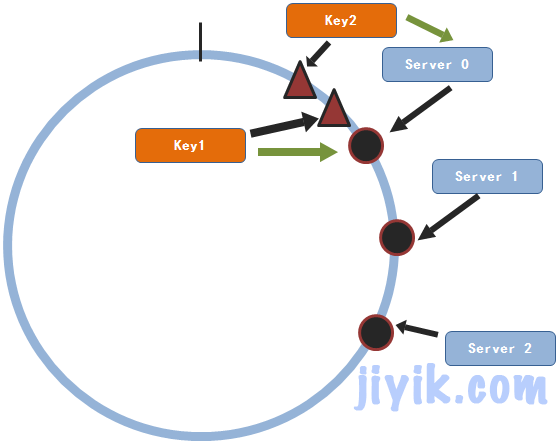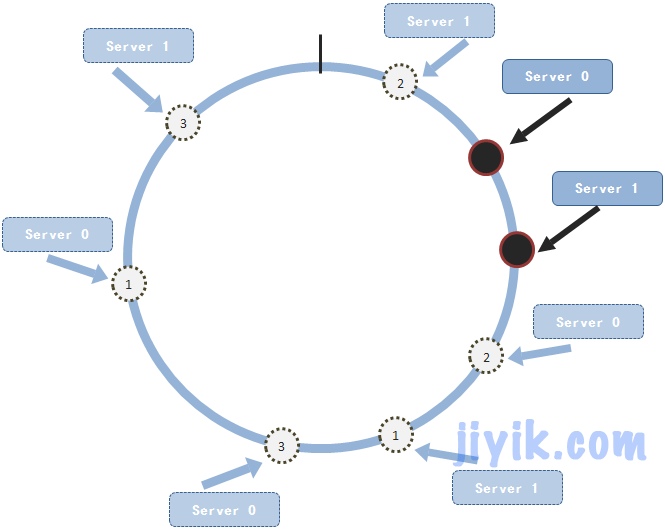``````\$nodes = array('192.168.5.201','192.168.5.102','192.168.5.111');
\$keys = array('onmpw', 'jiyi', 'onmpw_key', 'jiyi_key', 'www','www_key','key1');
//添加的变量  修改的地方
\$replicas = 160;  //每个节点的复制的个数
\$buckets = array(); //节点的hash字典
\$maps = array(); //存储key和节点之间的映射关系
/**
* 生成节点字典 —— 使节点分布在单位区间[0,1)的圆上
*/
foreach( \$nodes as \$key) {
//修改的地方
for(\$i=1;\$i<=\$replicas;\$i++){
\$crc = crc32(\$key.'.'.\$i)/pow(2,32);            // CRC値
\$buckets[] = array('index'=>\$crc,'node'=>\$key);
}
}
/*
* 根据索引进行排序
*/
sort(\$buckets);
/*
* 对每个key进行hash计算，找到其在圆上的位置
* 然后在该位置开始依顺时针方向找到第一个服务节点
*/
foreach(\$keys as \$key){
\$flag = false; //表示是否有找到服务节点
\$crc = crc32(\$key)/pow(2,32);//计算key的hash值
for(\$i = 0; \$i < count(\$buckets); \$i++){
if(\$buckets[\$i]['index'] > \$crc){
/*
* 因为已经对buckets进行了排序
* 所以第一个index大于key的hash值的节点即是要找的节点
*/
\$maps[\$key] = \$buckets[\$i]['node'];
\$flag = true;
break;

}

}
if(!\$flag){
//没有找到，则使用buckets中的第一个服务节点
\$maps[\$key] = \$buckets['node'];
}
}
foreach(\$maps as \$key=>\$val){
echo \$key.'=>'.\$val,"<br />";
}``````